## Convergence Improvement

The improvement of the convergence properties of a Series, also called Convergence Acceleration, such that a Series reaches its limit to within some accuracy with fewer terms than required before. Convergence improvement can be effected by forming a linear combination with a Series whose sum is known. Useful sums include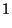(1)(2)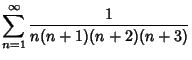(3)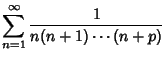(4)

Kummer's transformation takes a convergent series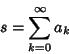(5)

and another convergent series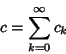(6)

with knownsuch that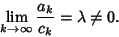(7)

Then a series with more rapid convergence to the same value is given by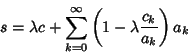(8)

(Abramowitz and Stegun 1972).

Euler's Transform takes a convergent alternating series(9)

into a series with more rapid convergence to the same value to(10)

where(11)

(Abramowitz and Stegun 1972; Beeler et al. 1972, Item 120).

Given a series of the form(12)

whereis an Analytic at 0 and on the closed unit Disk, and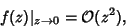(13)

then the series can be rearranged to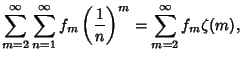(14)

where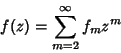(15)

is the Maclaurin Series ofandis the Riemann Zeta Function (Flajolet and Vardi 1996). The transformed series exhibits geometric convergence. Similarly, ifis Analytic in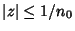for some Positive Integer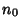, then(16)

which converges geometrically (Flajolet and Vardi 1996). (16) can also be used to further accelerate the convergence of series (14).

References

Abramowitz, M. and Stegun, C. A. (Eds.). Handbook of Mathematical Functions with Formulas, Graphs, and Mathematical Tables, 9th printing. New York: Dover, p. 16, 1972.

Arfken, G. Mathematical Methods for Physicists, 3rd ed. Orlando, FL: Academic Press, pp. 288-289, 1985.

Beeler, M.; Gosper, R. W.; and Schroeppel, R. HAKMEM. Cambridge, MA: MIT Artificial Intelligence Laboratory, Memo AIM-239, Feb. 1972.

Flajolet, P. and Vardi, I. Zeta Function Expansions of Classical Constants.'' Unpublished manuscript. 1996. http://pauillac.inria.fr/algo/flajolet/Publications/landau.ps.